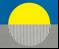APPENDIX A

Relativity and the Michelson-Morley Experiments

Compressible Photon Flow and the Results of Michelson-Morley Type Experiments

Bernard  A. Power1

August 2002

The interference fringe shifts that are always observed with experiments of the Michelson-Morley type have never been completely explained.  Special relativity and isothermal compressible flow theory both predict that no fringe shift should occur at all.  However, with nine degrees of energy partition, compressible theory applied to photon flows predicts the fringe shifts that are observed and which appear to reflect the earth’s basic motion in space.

PACS number(s): 03.30.+p.,  01.55.+b., 47.40.Dc.

The failure of a long series of classical experimental tests to detect any motion between the earth moving in its solar orbit and some sort of theoretical substratum or ether, using the Michelson interferometer to detect any anisotropy in the speed of light in space, eventually led to the abandonment of ether models and the universal adoption of the special theory of relativity.

With encouragement from Lorentz and Einstein among others, however, a continuing test of the Michelson-Morley  experiment by D. C. Miller  at Cleveland and at Mt. Wilson was carried on  until the mid -nineteen twenties.  All of these observations refuted the ether drift hypothesis.  But, equally, all of them yielded a definite small fringe shift upon rotation of the interferometer.  The tests of D.C. Miller were the most extensive, spanning several years of time and covering most of the epochs or seasons of the earth’s orbital year.  Instead of the full expected shift of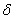= 1.12 fringe, he found a smaller average shift (= 0.122) which corresponded to a computed ether drift velocity of about 10 km/s rather than the expected earth’s orbital speed of 30 km/s.  The observations did show a very small seasonal and azimuth oscillation which, however, could never be put into any convincing relationship to physical effects.

Later versions of this type of interferometer experiment [3-7] generally reported considerably smaller average shifts, and, as these gradually came to be viewed as being simply statistical or environmental effects, inquiry was eventually dropped .

For the classical Michelson-Morley experiments, the expected fringe shift, calculated on the basis of Galilean addition of orbital velocity to the speed of light, is given by= 2 l (Vo /co)2 /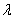(1)

Here l is the optical path length (32 meters in the Miller apparatus), co is the speed of light in space, Vo   is the mean orbital speed of the earth taken as 30 km/s, andthe wavelength of light used by Michelson-Morley and by Miller is 5.7 x 10-7 m.  The expected fringe shift from Eq.1 was 1.12.  A zero fringe shift was to be expected from special relativity, and eventually the smaller fringe shifts (0.122 average) which were always observed were also taken as being effectively zero. However, there is a third possibility, which is that of compressibility in the energy flow.  Lorentz  speculated that compressibility might somehow be a factor in reconciling Miller’s results with the apparently necessary properties of the hypothetical ether then under discussion: He said  …in the case of Planck’s modification of Stokes theory.  A further possibility would be a compressible ether.  This would remove even the necessity of having an irrotational ether.

If, however, we apply compressibility, not to an ether, but instead  to an energetic field  - for example a compressible fluid flow, a compressible gravitational field, a compressible ‘vacuum’ field, or a compressible photon flow - we have the basic steady state  energy equation [11-12]

c2 = co2 - V2 /n                                                                       (2)

Here the mass m is taken as being unity, as is usual in hydrodynamics; co for a photon flow is the static compression wave speed equal to 3 x 108 m/s (when V the uniform relative motion is zero); c is the reduced wave speed under any uniform relative flow V; n is the number of degrees of freedom of the field, that is the number of ways the energy of the system is equally divided.  For one-dimensional motions (n = 1) the right hand side of Eq. 2 becomes (c0 + V)( c0 – V) which is also the going-and-coming addition of velocities used in deriving the classical fringe shift Eq. (1).  Since these predicted shifts are nine or ten times too large, attention historically then turned to finding a theory that predicts  zero fringe shifts instead.  In the present theory we can rearrange  Eq. (2)  with n = 1 to get

c/c0 = [1 - (V/co)2]1/2                                                                                                        (3)

and the right hand side now becomes, when inverted, identical to the Lorentz/Fitzgerald contraction factor, which, if it is arbitrarily applied to shorten the length of  the interferometer arm in the direction of V,  will then exactly cancel the reduction  in the  speed of light caused by V and give a zero fringe shift.  A second way to employ compressibility to get a zero shift  would be to take n = ∞ so that in Eq. (2)  V2/n becomes zero, and c is always equal to c0;  thermodynamically  this would require  isothermal flow.

There is also, however, the possibility of having a compressible photon flow with multiple degrees of energy partition. When this option is explored there is at once an improvement in explaining the observed residual fringe shifts.  However, there is still no really good fit obtained until we take n = 9.  For steady flow the fringe shift Eq. (1)  becomes= 2 l (Vo /co)2 /9(4)

Relationship (4) greatly changes the prediction of fringe shifts since these now become only one ninth of the classical expectations from Eq.(1), while classical ether drift velocities associated with any observed shift become three times larger. For example, Miller in his day expected a fringe shift of 1.12 from Eq. (1), whereas now, with compressible flow and n = 9 assumed, we find that the expected fringe shift becomes 0.125 which is close to  what Miller observed (2), his average for  the four seasons being 0.122. The flow velocity now becomes  three times larger, so that= 0.122 corresponds not to Miller’s computed 10 km/s but to √9 x 10 km/s or 30 km/s  which is the expected orbital speed.

The small velocity changes associated with the earth’s cosmic motions are:  (a) the change in the earth’s orbital velocity ΔVo from perihelion on January 3 to aphelion on July 3  of approximately 498 m/s over the half solar year;  (b) changes in the expansion and contraction velocity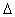Vr  of the earth’s orbital radius  between perihelion and aphelion, which amount to about 322 m/s over the 180 days, and   (c) the tangential velocityVrev   associated with the  diurnal  revolution of the earth on its axis,  which is about 385 m/s at 34° N, the latitude of Pasadena and Mount Wilson. These are not considered here.

In any assessment of the results of the various interferometer tests, it should  noted that the observed data are fringe shifts, except where masers were used, in which case the basic observations are a beat frequency or frequency shift. In the classical literature the test results are commonly expressed as ether drift velocity which is just the basic fringe shift observation converted to an equivalent drift velocity using Eq. (1).  Early experiments concentrated on attempts to detect the orbital speed of the earth namely 30 km/s.  Later, when only about one third of this velocity was found, the approach changed fundamentally; the fairly large fringe shifts observed at each rotation through 90° were discarded, since they were too small to be explained by classical ether drift theory, and efforts were directed instead at trying to find very small changes in the fringe shifts at different times of the day so as to sample any effect of the changing orientation of the instrument in space as the earth turned on its axis.  However, as we shall see, with compressible flow and n = 9, the large discarded basic fringe shifts observed at each 90 degree turn  substantially match  the  orbital speed of  30 km/s that was earlier thought to be lacking.

Turning now to other tests than those of Miller, there is first the original Michelson-Morley test in 1887 at Cleveland [1,2] that used an optical path length of 1100 cm and light with wavelength of 5.7 x 10-7 m. They reported an equivalent ether drift velocity of not more than 5 km/s to 7.5 km/s in 6 hours of testing over four days in July. Based on compressibility considerations with n = 9 this would corresponds to cosmic motions of  about 15  to 22.5 km/s.   Miller later recalculated the results using a harmonic analyzer to retrieve the ether drift periodicity (i.e. the effect which is periodic in each 180 degree turn of the interferometer) and got 8 to 8.8 km/s, the corresponding compressibility calculation then giving  24 to 26.4 km/s (n = 9).

Morley & Miller  in 1904, also at Cleveland,  used an optical  length of 3220 cm, and in October observed a drift of 8.7 km/s (fringe shift 0.12) corresponding to about 26.1 km/s in compressibility theory with n = 9.

Michelson, Pease and Pearson  used an instrument similar to that of Miller, with an optical length of 25.9 m, which was installed at Mount Wilson in a basement room having small temperature or pressure variations. They reported their results to be an ether drift velocity not greater than 6 km/s, which corresponds in compressibility theory to 18 km/s.

Kennedy and Thorndike  (1929-30) at Pasadena used an ingenious interferometer with one arm longer than the other by only 0.16 m. They used light of wavelength 5.461 x 10-7 m. Their small instrument was completely enclosed in an inert gas and could be maintained at a near constant temperature over long periods of time. The instrument was not rotated but, instead, observations were taken at a fixed azimuth orientation daily over a full year, the fringe shifts being photographically recorded.  For the case of the diurnal observations their average fringe shift was 0.000212, which, from Eq. (4) with n = 9, gives an orbital velocity of 17.1 km/s. They also reported an annual orbital effect of 10 ± 10 km/s, corresponding to 30 ± 30 km/s with n = 9, a result in which they did not express much confidence

Illingworth  at Pasadena used an improved instrument, originally designed and used by Kennedy, which had a much shorter optical path length ( 2 meters)  It was kept in an isothermal room.  It was not rotated continuously but was turned intermittently through 90 degrees, going from either north to west or northwest to southwest. Observations were carried out for a period of 10 days in early July.  Illingworth  observed a basic fairly large shift  at each 90° rotation, which in his  calculations he discarded, presumably because  on classical fringe theory it would not have given the orbital speed of 30 km/s but only about a third as much. He does not explicitly give the magnitude of this discarded rotational  fringe shifts  except for a one hour period at 11 A.M on July 9, where he tabulated  a weight difference of  4.7 units  at each turn, and at 1/500 fringe shift  per weight,  this gives a fringe shift of 0.0094 with each 90° turn of the instrument.  From Eq. (4) with n = 9  this corresponds to 32.6 km/s which is about 10% higher than the aphelion value of 29.557 km/s on July 3, but of course this estimate  rests on only the one 11 A.M. observation. The small variations in the basic large background shift which was observed  at intervals over the day  ranged from a maximum of 0.00036 at 5 A.M to 0.000008 at 11 A.M., 0.000082 at 5 P.M. and 0.00034 at 11 P.M.

Joos  (1930) at Jena used an elegant instrument with an optical length of  21 meters. His instrument was sealed and kept at a near constant temperature. His observations were made during twenty one days in May.  Like Illingworth he observed a large fringe shift at each rotation but searched only for variations on this basic larger background during the day.  He does not give the magnitude of the larger rotational shift, but from inspection of the fringe photographs reproduced in his paper it would appear to be about 0.1 to 0.08 fringe per rotation. From Eq. (6a) shift of  0.083 would correspond to 29.557 km/s, which is the orbital speed at aphelion on July 3. He reported an average fringe shift of 0.001 for the small diurnal  variations with changing orientation.

There were two tests of space anisotropy and special relativity carried out using masers shortly after they became available. The basic observations in these experiments were beat frequency shifts upon rotation of the instrument, this quantity being proportional either to uV/c02 or (V/c0)2.  In reassessing these tests with compressible flow theory and n = 9, these two velocity ratios are to be divided by 9.

1) Cedarholm and Townes  (1959) used an ammonia maser to look for an ether drift effect in the Doppler shift.  They compared the frequencies of two such masers with their opposing beams run in parallel, and when the apparatus was rotated through 180°. Their tests were run at intervals over a year.  The expected beat shift f, based on an ether drift effect, was f = [4uV/co2  where V = 30 km/s, u the speed of the ammonia molecules is 600 m/s, and ν  the frequency of the excited molecules is 2.387 x 1010 /s. The expected beat shift was 19.8 cps, so that the actual expected frequency shift was about 10cps. The observed shift was only 1.08 ± 0.02 cps. Their conclusion was, since the observed shift of 1.08 was far less than the predicted 10 cps, that the speed of light was thereby shown to be unaffected by the orbital motion of the earth in space of 30 km/s.

However, if compressible flow with  n = 9  is included, then the predicted  frequency shift becomes instead  f = 10/9 = 1.11 cps , which agrees well with the observed value of 1.08.

(2) Jaseja, Javan, Murray and Townes  (1964), using two rotating masers positioned at right angles to one another, tested for the ether drift second order effect in  (V/c)2.  They reported on a short six hour test in January.  Upon rotation through 90° they got a basic, repeating frequency shift of 275 kc/s plus a variation of not more than 3 kc/s. They discarded the observed repeatable frequency shift on rotation of 275 kc/s as presumably due to magnetostriction, and concluded that there was no evidence of any frequency shift effect arising from the from the earth’s orbital motion  of 30 km/s

Their expected frequency shift equation was 2Δν ≈ (V/co)2 , which for V = 30 km/s gives 3000 kcs expected frequency shift on rotation. Only about 275kcs shift was observed and was discarded.  However, when the compressibility parameter n is included, we get 2Δν ≈ (V/co)2 /9 which gives 3000/9 = 333 kcs predicted versus 275 observed or only about 20%  high. Expressed in relative motion terms this corresponds to about 27.2 km/sec.

Compressible photon flow theory is self-consistent and moreover it matches the experimental data, thereby fulfilling the two essential requirements for viability.  We can then, from this new standpoint, evaluate classical  Galilean addition of velocities, the Lorentz/Fitzgerald contraction factor and the special relativity theory of  Lorentz transformations.

Classical or Galilean addition of velocities uncritically extended the addition of mass particle velocities to the case of addition of particle velocity to the wave speed of light c in the formulation of the classical fringe shift equation for the Michelson-Morley experiment. Fortuitously, the two-way going-and-coming design of the experiment involves forming the quantity ( c2 - V2) = (c + V ) (c- V) which, if c is identified with the static speed wave speed co, agrees  correctly with the compressible flow Eq. (2) for n =1. It is important to realize that with n = 1 all of the velocity adds to or subtracts from the wave speed c, whereas with n larger than unity only a fraction of V adds  to or subtracts from c  which gives   (c + V/√n)( c - V/√n) = (co2 - V2/n).

The classical formulation with n =1 predicted the impossibly large fringe shifts which posed a major problem to the science of the day. The Lorentz/Fitzgerald solution   was not just to reduce the fringe shift but to eliminate it entirely. This was accomplished by inserting into the velocity transformations an ad hoc reduction factor [1- (V/co)2]-1/2 which simply cancelled the large shift completely.

The special theory  of relativity succeeded in putting the ad hoc formulation on a theoretical basis by assuming, first, the classical Galilean addition of particle and wave velocities as before, and second by postulating the constancy of the speed of light in all relatively moving inertial coordinate systems. When this is done the Lorentz/Fitzgerald contraction factor emerges automatically. The price of an apparent inconsistency  was eventually felt to be justified since the unacceptably large fringe shift predictions were now eliminated. On compressible flow theory, such an addition of the entire relative velocity V to the  wave speed c would be interpreted as being an adiabatic photon flow with n = 1, while the postulated constancy of c = c0 would necessarily be seen as an isothermal flow (n = ∞); the two assumptions taken together now become physically inconsistent.

The development of compressible theory in the last half-century permits the alternative solution outlined here, which not only explains the partial success of previous attempts, but which, instead of incorrectly eliminating the fringe shift entirely, reduces it to the experimentally  observed values by postulating a relative photon flow  with n = 9.

The transformations between relatively moving inertial coordinate systems in the new theory are made by replacing the static speed of light co with the reduced local speed of light c required from Eq. (2), by computing c = co [1- 1/9(V/co)2]1/2 = co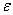.  Alternatively, if desired or more familiar, the compressibility could be ignored, with the wave speed arbitrarily taken at  the static speed of light co  ( 3 x 108 m/s)  in all coordinate systems as before, but then the  factor γ of special relativity theory  (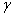= [1- (V/co)2]-1/2) must be replaced by the factorwhere 1/= c/co = [1- (1/9)(V/co)2]1/2 in the transformation equations. If this computation method is chosen, the space and time coordinates and the various physical quantities must then be altered to:= l'/lo = dto/dt' = m'/mo,  etc.

In the case of a material gas, the energy partition is among random kinetic motion of molecules in three space dimensions plus perhaps rotation and vibration, and these random motions give rise to pressure and compressibility.  For photons, however, there are no obvious equivalents to thermodynamic pressure, random kinetic motion, and so on, and therefore the precise physical nature of a photon flow that could give rise to the observed agreement with compressible flow theory remains to be established. However, it does appear possible that three space dimensions, each with associated states, plus vibration states  might provide the nine-dimensional energy partition required to explain the results summarized in Table 1.

Table 1. Observed interference fringe shifts and corresponding computed flow velocities.

---------------------------------------------------------------------------------------------------------

Observer               Observed            “Ether drift”   Compressible flow (n = 9)

fringe shift             velocity V a       Orbital

()                     (km/s)             velocity V b

(km/s)

---------------------------------------------------------------------------------------------------------

Michelson-Morley    0.01                      5 - 7.5                   15 - 22.5

(1887) Cleveland    (Miller revision)     8-  8.5                   24 - 25.5

Morley & Miller      0.09                      8.7                        26.1

(1905) Cleveland

Miller                    0.122 (avg)            10.05                    30.1

(1925-6) Mt. Wilson

Michelson et al.       0.01 (max)             ≤6                        ≤18

(1929) Mt. Wilson

Kennedy-Thorndike    0.000212 (daily)                                17.0 c

(1929-30)              (annual)                 10 ± 10                 30 ± 30

Illingworth               0.0094        (rotational)                       32.6 c

Joos                       0.083 (est.) (rotational)                       29.56 c

(1930) Jena            0.001        (orientational)

---------------------------------------------------------------------------------------------------------

Cedarholm & Townes         Maser          1.08 ± 0.02 cps   (30 km/s ± 556 m/s)

(1959) New York

Jaseja et al.                       Maser           275 ± 3 kc/s        27.2 km/s                                                        (29

(1964) Round Hill, Mass.

---------------------------------------------------------------------------------------------------------

a Computed from Eq. 1;      b V = (‘ether drift’)x√9;          V computed from Eq. 4;

References

   A.A. Michelson and E.W. Morley,  Am. J. Sci. 34, 333 (1887).

   D.C. Miller,  Rev. Modern Physics, 5, 203 (1933).

   H.A. Lorentz,  Astrophys. J. 68, 395 (1928).

   K.K Illingworth,  Phys. Rev. 30, 692 (1927).

   A.A. Michelson, F.G. Pease, and  F. Pearson,  Nature, 123, 85 (1929).

   G. Joos,  Ann. d. Physik, 7, 385 (1930).

   R.J.  Kennedy and  E.M Thorndike,  Phys. Rev. 42, 400 (1932).

   J. P. Cedarholm and  C.H. Townes,  Nature, 184, 1350-51 (1959).

   T.S. Jaseja,  Javan,  J. Murray, and C.H. Townes,  Phys. Rev. 133, A1221 (1964).

 R.S. Shankland, et al.  Rev. Modern Physics. 27, 167-178 (1955).

 A.H. Shapiro,  The Dynamics and Thermodynamics of Compressible Fluid Flow. (John Wiley & Sons, New York, 1954).

 R. Courant and K.O. Friedrichs,  Supersonic Flow and Shock Waves. (Interscience, New York, 1948).

Note:  For a review of latest laser/maser/oscillator tests of Lorentz invariance  see Main Page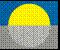MAIN PAGE/